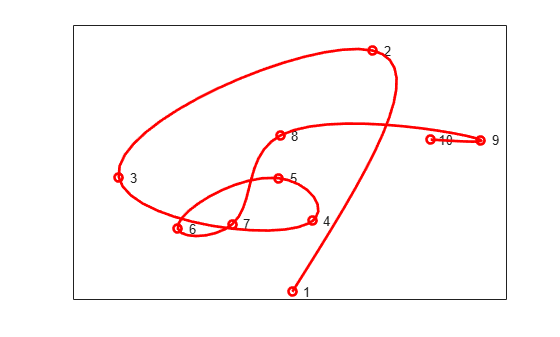# Constructing Spline Curves in 2D and 3D

This example shows how to use the `cscvn` command from Curve Fitting Toolbox™ to construct cubic spline curves in two and three dimensions.

### Selecting the Points

This example will show how to draw a smooth curve through a list of points, in the order in which they occur. First, we select some random points in the plane, and store them in a matrix, one point per column.

```npts = 10; xy = [randn(1,npts); randn(1,npts)]; plot(xy(1,:),xy(2,:),'ro','LineWidth',2); text(xy(1,:), xy(2,:),[repmat(' ',npts,1), num2str((1:npts)')]) ax = gca; ax.XTick = []; ax.YTick = [];```### Connecting the Points

Next, construct the curve using the `cscvn` command and plot it using `fnplt`.

```hold on fnplt(cscvn(xy),'r',2) hold off```You could also use the `getcurve` command if you wanted to input the list of points interactively.

### 3-D Spline Curves

It's just as easy to create spline curves in three dimensions. This time, we'll do something less random. First, we generate the points.

```npts = 13; t = linspace(0,8*pi,npts); z = linspace(-1,1,npts); omz = sqrt(1-z.^2); xyz = [cos(t).*omz; sin(t).*omz; z]; plot3(xyz(1,:),xyz(2,:),xyz(3,:),'ro','LineWidth',2); text(xyz(1,:),xyz(2,:),xyz(3,:),[repmat(' ',npts,1), num2str((1:npts)')]) ax = gca; ax.XTick = []; ax.YTick = []; ax.ZTick = []; box on```### Connecting the Points

Here is the 3D spline curve through these points provided by `cscvn`. By appending the first point to the end of the list, we get a smooth closed curve.

```hold on fnplt(cscvn(xyz(:,[1:end 1])),'r',2) hold off```